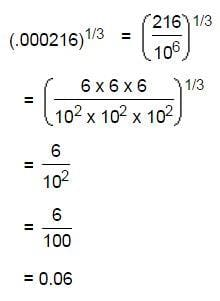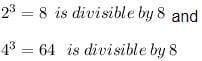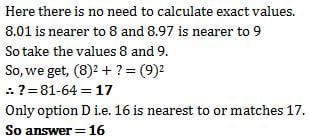Test: Cubes

# Test: Cubes

Test Description

## 10 Questions MCQ Test CSAT Preparation for UPSC CSE | Test: Cubes

Test: Cubes for DSSSB TGT/PGT/PRT 2022 is part of CSAT Preparation for UPSC CSE preparation. The Test: Cubes questions and answers have been prepared according to the DSSSB TGT/PGT/PRT exam syllabus.The Test: Cubes MCQs are made for DSSSB TGT/PGT/PRT 2022 Exam. Find important definitions, questions, notes, meanings, examples, exercises, MCQs and online tests for Test: Cubes below.
Solutions of Test: Cubes questions in English are available as part of our CSAT Preparation for UPSC CSE for DSSSB TGT/PGT/PRT & Test: Cubes solutions in Hindi for CSAT Preparation for UPSC CSE course. Download more important topics, notes, lectures and mock test series for DSSSB TGT/PGT/PRT Exam by signing up for free. Attempt Test: Cubes | 10 questions in 10 minutes | Mock test for DSSSB TGT/PGT/PRT preparation | Free important questions MCQ to study CSAT Preparation for UPSC CSE for DSSSB TGT/PGT/PRT Exam | Download free PDF with solutions
 1 Crore+ students have signed up on EduRev. Have you?
Test: Cubes - Question 1

### The cube root of .000216 is:

Detailed Solution for Test: Cubes - Question 1Test: Cubes - Question 2

### Cube root of 0.064 is

Detailed Solution for Test: Cubes - Question 2

0.064 = 64/1000= 4^3 / 10^3 =(4/10)^3= (2/5)^3

taking cube root of 0.064= 2/5=0.4

Test: Cubes - Question 3

### The cube of any multiple of 2 is always divisible by

Detailed Solution for Test: Cubes - Question 3

Cube of multiple of 2 is divisible by 8
eg:Test: Cubes - Question 4

What is the smallest number by which 3600 be divided to make it a perfect cube?

Detailed Solution for Test: Cubes - Question 4

Prime factorise 3600.

You will get 3600 = 2 x 2 x 2 x 2 x 3 x 3 x 5 x 5

Now group the numbers into groups of 3.

You will get 1 group of 2 and remaining numbers i.e. 2,3,3,5,5

Now multiply these extra numbers

You will get 2 x 3 x 3 x 5 x 5 = 450

So, 3600 divided by 450 = perfect cube (8)

Test: Cubes - Question 5

Cube of all odd natural numbers is

Detailed Solution for Test: Cubes - Question 5

Odd, Any odd number to power 3 will have unit digit odd

Ex.  7^3=3. 49^3=9.

Test: Cubes - Question 6

(8.01)2 + ? = (8.97)2 What will approximately come in place of question mark?

Detailed Solution for Test: Cubes - Question 6Test: Cubes - Question 7

The smallest number by which 5400 must be multiplied so that it become a perfect cube, is

Detailed Solution for Test: Cubes - Question 7

Find prime factors of 5400

5400=2×3×3×3×2×2×5×5

If we group them in the group of 3

5400=(2×2×2)×(3×3×3)×5×5

Here to make group of 3 for 5

We have to multiply 5400 by 5.

Test: Cubes - Question 8

Cube of all even natural numbers is

Test: Cubes - Question 9

Cube of a natural number will never end with the digit

Detailed Solution for Test: Cubes - Question 9

There'd no such digit which can't end in a cube of a natural number. Hence a cube of a natural number can end with any digit.
1³= 1 (ends with 1)
2³= 8 (ends with 8)
3³= 27 (ends with 7)
4³= 64 (ends with 4)
5³= 125 (ends with 5)
6³= 216 (ends with 6)
7³= 343 (ends with 3)
8³= 512 (ends with 2)
9³= 729 (ends with 9)
0³= 0 (ends with 0)
Hence the last digits are 0,1,8,7,4,5,6,3,2,9
Therefore we see every digits are last digits of these cubes. Hence a cube of a natural number can end with any digit.

Test: Cubes - Question 10

Cube of 0.2 is

Detailed Solution for Test: Cubes - Question 10

0.2×0.2×0.2=0.008

## CSAT Preparation for UPSC CSE

72 videos|64 docs|92 tests
 Use Code STAYHOME200 and get INR 200 additional OFF Use Coupon Code
Information about Test: Cubes Page
In this test you can find the Exam questions for Test: Cubes solved & explained in the simplest way possible. Besides giving Questions and answers for Test: Cubes, EduRev gives you an ample number of Online tests for practice

## CSAT Preparation for UPSC CSE

72 videos|64 docs|92 tests